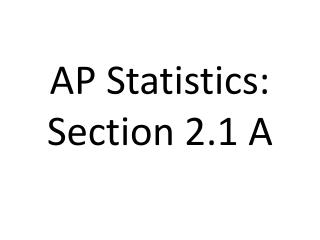DownloadDownload PresentationAP Statistics: Section 2.1 A

# AP Statistics: Section 2.1 A

Télécharger la présentation## AP Statistics: Section 2.1 A

- - - - - - - - - - - - - - - - - - - - - - - - - - - E N D - - - - - - - - - - - - - - - - - - - - - - - - - - -
##### Presentation Transcript

1. AP Statistics: Section 2.1 A

2. Measuring Relative Standing: z-scoresA z-score describes a particular data value’s position in relation to the rest of the data.

3. In particular, a z-score tells us how many standard deviations a particular score is above or below the mean.

4. Since a z-score is in standard deviation units, converting a data to a z-score is called____________ standardizing.

5. If x is an observation from a distribution that has known mean and known standard deviation , then the standardized value of x is:

6. Observations larger than the mean have ________ z-scores, whereas observations smaller than the mean have ________ z-scores. positive negative

7. Example 1: Kerry earned a 93 on the Chapter 1 test and Norman earned a 72. The median and mean for the class were both 80 and the standard deviation was 6.07. Determine, and interpret their respective z-scores. Kerry’s score is 2.14 stand. dev. above the mean Norman’s score is 1.31 stand. dev. below the mean.

8. Example 2: Kerry earned a scored of 82 in his calculus class. The class had a mean of 76 and a standard deviation of 4. Did he do worse on this test than on his stats test? Compared to the class, Kerry did worse on his calculus test, because he is only 1.5 stand. dev. above the mean.

9. Measuring Relative Standing: Percentiles

10. In Chapter 1, we defined the pth percentile of a distribution as the value that has p percent of the observations fall at or below it. Some people define the pth percentile of a distribution as the value with p percent of observations below it. Using this definition, it is impossible for an individual to fall at the _________ percentile. That is why you never see an ACT score reported above the 99th percentile.

11. As long as you use a definition for percentile that is common use, you will use receive full credit on the AP exam.

12. Example 3: Jenny’s score on her stats test was the 3rdhighest score in the class. If there are 25 students in the class, determine her percentile.

13. Example 4: Norman’s score was the 2nd lowest score. Determine his percentile.

14. There is no simple method to convert a z-score to a percentile. The percentile depends on the shape of the distribution. In a perfectly symmetrical distribution, the mean _______ the median. Thus, in a perfectly symmetrical distribution a z-score of 0 will equal the ______ percentile. But, in a left-skewed distribution, where the mean is ______ than the median, this observation will be somewhere ________ the 50th percentile. equals 50th less below

15. There is a theorem that describes the percent of observations in any distribution that falls within a specified number of standard deviations of the mean. It is know as Chebyshev’s Inequality.

16. In any distribution, the percent of observations falling within k standard deviations of the mean is

17. Example 5: Complete the following table to determine the percent of observations in any distribution that must fall within k standard deviations of the mean.

18. Chebyshev’s Inequality gives us some insight into how observations are distributed within distributions. It does not help us determine the percentile corresponding to a given z-score. For that, we need more advanced models known as ______________. density curves